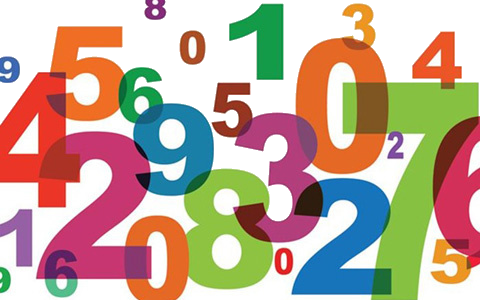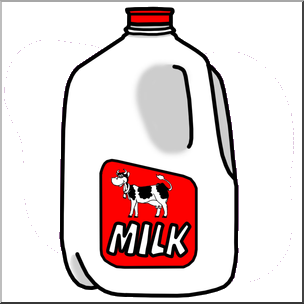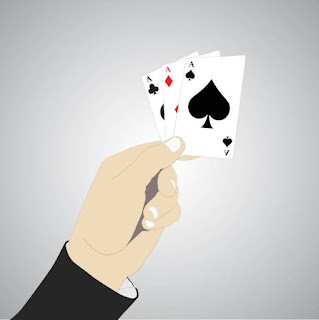### Logical Thinking Puzzles# Blue Fishes

There are 200 fishes in a pond. 99% fishes are blue. How many blue fishes should be removed to make the percentage of blue fishes in to 98%?

Hint: 2 is not the answer.

First, why '2' is not the right answer? If you think 198 is the initial number of blue fishes and if 2 blue fishes are removed, then there will be 196 blue fishes and hence in terms of percentage it is 98. But its wrong, since the total fishes count also decreased to 198, if you just remove 2 blue fishes alone. So the percentage of blue fishes are (196/198) × 100 = 98.99% Its not 98% its almost 99%.
In simple, 198 blue fishes and 2 other colored fishes are there initially. And if you remove 100 blue fishes, then 98 blue fishes with 2 other colored fishes. So, the percentage of blue fishes becomes 98.
Answer: 100 blue fishes should be removed.# Tennis Match

Antony and Benjamin played tennis against each other. Its a bet game and \$1 for the winner.
After several games, Antony won \$3 and Benjamin won \$5. How many games did they play?

Benjamin lost 3 games to Antony, but won \$5 finally. So, to overcome \$3 loss, he played 3 more games and again to win \$5, he played 5 extra games.
So, in total 3+3+5 = 11 games were played.# Number puzzle

The sum and the difference between a two-digit number and the number obtained by interchanging the positions of the digits is 110 and 54 respectively. What is the two-digit number?

It can be solved by simple algebraic method. Assume the ten’s digit as 10x and the unit digit as ‘y’.
So (10x + y) + (10y + x) = 110 → 1
(10x + y) – (10y + x) = 54 → 2
Simplifying equation 1, then 11x + 11y = 110 → 3
Similarly simplifying equation 2, then 9x – 9y = 54 → 4
Divide the equation 4 by 9, then x – y = 6
Or x = 6 + y → 5
Substitute equation 5 in 3 for ‘x’.
11 × (6+y) + 11y = 110
66 + 11 y + 11y = 110
22 y = 44
Or y = 2
Hence from equation 5, value of x = 8
And the two digit number is (xy) 82.# Find the age

A man is 24 years older than his son. In 2 years, his age will be twice the age of his son. What is the present age of his son?

Assume present age of the son as ‘a’.
And assume father’s present age as 'b' and b = (a + 24).
After two years:
2 × (a+2) = (b+2)
2 × (a+2) = (a+24+2)
2a + 4 = a + 26
(2a – a) = 26 – 4
a = 22 years.# Candies

A shop is selling 3 candies for Rs. 1 and if you return 3 of the candy wrappers you will get 1 candy free. How many candies will you get for Rs. 45 and how many wrappers will be left?

For Rs. 45 you will get (45×3 =) 135 candies.
Then return 135 wrappers. For the return of every 3 wrappers, you will get 1 candy. And for 135 wrappers, you will get 135/3 = 45 candies.
Then for these 45 wrappers, you will get 45/3 = 15 candies.
For these 15 wrappers, you will get 15/3 = 5 candies.
Eat 3 candies from this 5 and return just 3 wrappers. Again you will get 1 candy for this.
Out of 5 candies 2 is remaining and hence 2 + 1 = 3 candies.
Eat the 3 candies and return the 3 wrappers and get 1 more candy.
In total, 135 + 45 + 15 + 5 + 1 + 1 = 202 candies and just 1 wrapper will be left.# Milk & Water

150 L of milk contains 20% water. How much water should be added to make the water content as 25%?

100 L milk contains 20 L water.
∴ 150 L milk contains (150 × 20)/ 100 = 30 L water
In simple it contains, 120 L milk and 30 L water.
If it contains 25% water, then 75% milk should be there.
Or, [120 / (120 + x)] × 100 = 75,
where, ‘x’ is total volume of water in milk with 25 % water.
(120 + x) = (120 × 100) / 75 = 160.
x = 160 – 120 = 40 L.
Already 30 L water is there, and hence (40 – 30 =) 10 L water should be added to make water as 25%.
And hence the final volume will be 160 L.# 3 Aces

From a standard deck of 52 cards, 3 cards are drawn randomly together. What is the probability that all the 3 cards are aces?